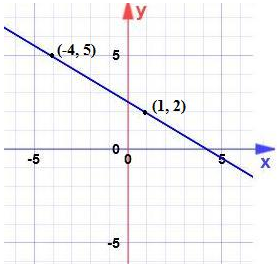Monday, September 27, 2021
Home > Question and Answer > Straight Lines Question And Answer Set 1

# Straight Lines Question And Answer Set 1# Straight Lines Question and Answer Set 1

Hi students, welcome to Amans Maths Blogs (AMB). On this post, you will get the Straight Lines Question and Answer Set 1 is the collection of some important questions. Practice these questions for SSC CGL CHSL CAT NTSE NSEJS exams etc. It will help you to practice the questions on the topics of maths as straight lines based questions of coordinate geometry.

Straight Lines Question and Answer Set 1: Ques No 1

The equation of the straight line shown in the diagram isOptions:

A. y = (-5/3)x + 13/5

B. y = (5/3)x + 5/13

C. y = (-3/5)x + 13/5

D. y = (3/5)x + 5/13

Straight Lines Question and Answer Set 1: Ques No 2

The locus of point P if P is equidistant from points A(3, 4) and B(5, -2) is

Options:

A. x – 3y = 1

B. x + 3y = -1

C. x – 3y = -1

D. x + 3y = 1

Straight Lines Question and Answer Set 1: Ques No 3

The equation of the line which is perpendicular to the lineand passes through the point (1,1) is

Options:

A. x + 2y – 1 = 0

B. x – 2y + 1 = 0

C. x – 2y – 1 = 0

D. x + 2y + 1 = 0

Straight Lines Question and Answer Set 1: Ques No 4

The lines 4x – ky = 6 and 6x + 3y + 2 = 0 are perpendicular. The value of k is

Options:

A. -6

B. 8

C. 5

D. -3

Straight Lines Question and Answer Set 1: Ques No 5

The equations of two equal sides of an isosceles triangle are 7x – y + 3 = 0 and x + y – 3 = 0 and the third side passes through the point (1, 10). The equation of the third side is

Options:

A. x + 3y + 31 = 0

B. 3x + y – 7 = 0

C. x + 3y – 31 = 0

D. 3x + y + 7 = 0

Straight Lines Question and Answer Set 1: Ques No 6

A ray of light coming from the point (1, 2) is reflected at a point A on the x-axis and then passes through the point (5, 3). The coordinate of the point A is

Options:

A. (5/13, 0)

B. (13/5, 0)

C. (6, 1)

D. (3, -5)

Straight Lines Question and Answer Set 1: Ques No 7

If the coordinate of the middle point of the portion of line intercepted between coordinate axes (3, 2), then the equation of the line is

Options:

A. 2x + 3y = 12

B. 3x + 2y = 12

C. 4x – 3y = 6

D. 5x – 2y = 10